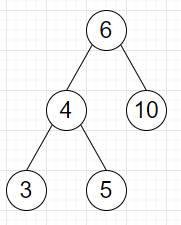# Program to find sibling value of a binary tree node in Python

Suppose we have a value k and a binary search tree, here each node is either a leaf or contains 2 children. We have to find the node containing the value k, and return its sibling's value.

So, if the input is likek = 4., then the output will be 10.

To solve this, we will follow these steps −

• Define a function util() . This will take root, k, ans

• if left of root is not null and right of root is not null, then

• return

• if k > value of root, then

• if value of right of root is same as k, then

• insert value of left of root at the end of ans

• return

• otherwise,

• util(right of root, k, ans)

• if k < value of root, then

• if value of right of root is same as k, then

• insert value of right of root at the end of ans

• return

• otherwise,

• util(left of root, k, ans)

• From the main method, do the following −

• ans := a new list

• util(root, k, ans)

• return ans

Let us see the following implementation to get better understanding −

## Example

Live Demo

class TreeNode:
def __init__(self, data, left = None, right = None):
self.val = data
self.left = left
self.right = right

def util(root, k, ans):
if root.left is None and root.right is None:
return
if k > root.val:
if root.right.val == k:
ans.append(root.left.val)
return
else:
util(root.right, k, ans)
if k < root.val:
if root.left.val == k:
ans.append(root.right.val)
return
else:
util(root.left, k, ans)

class Solution:
def solve(self, root, k):
ans = []
util(root, k, ans)
return ans

root = TreeNode(6)
root.left = TreeNode(4)
root.right = TreeNode(10)
root.left.left = TreeNode(3)
root.left.right = TreeNode(5)
ob1 = Solution()
print(ob1.solve(root, 4))

## Input

root = TreeNode(6)
root.left = TreeNode(4)
root.right = TreeNode(10)
root.left.left = TreeNode(3)
root.left.right = TreeNode(5)
4

## Output

10

Updated on: 21-Oct-2020

443 Views